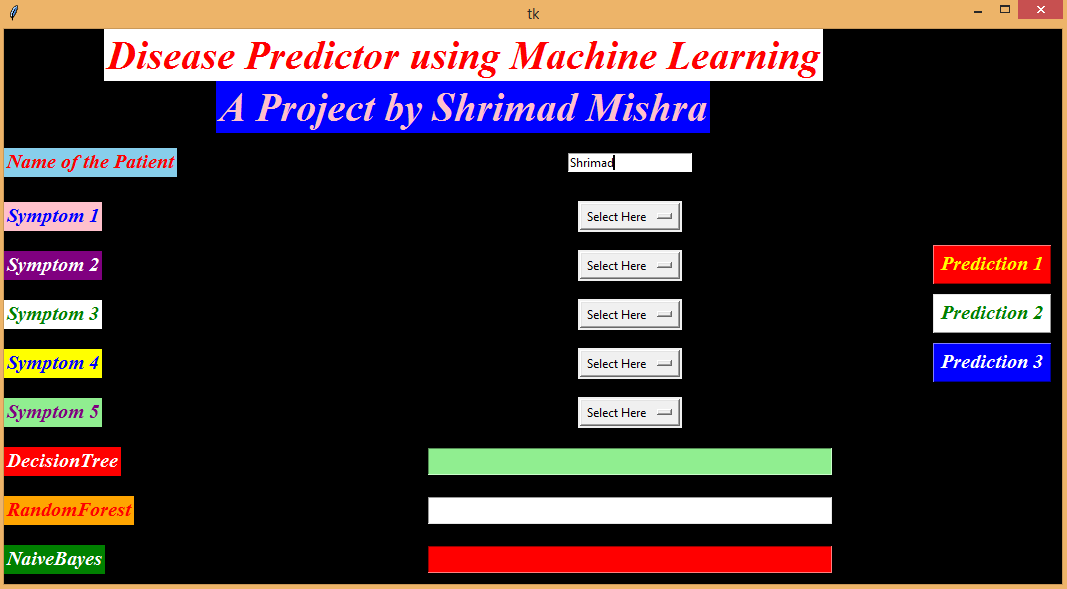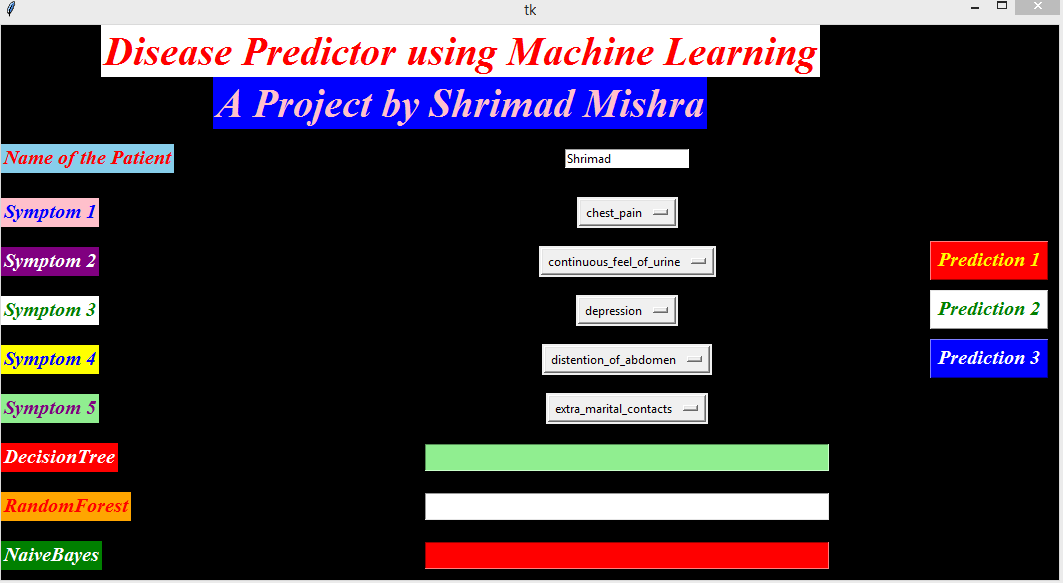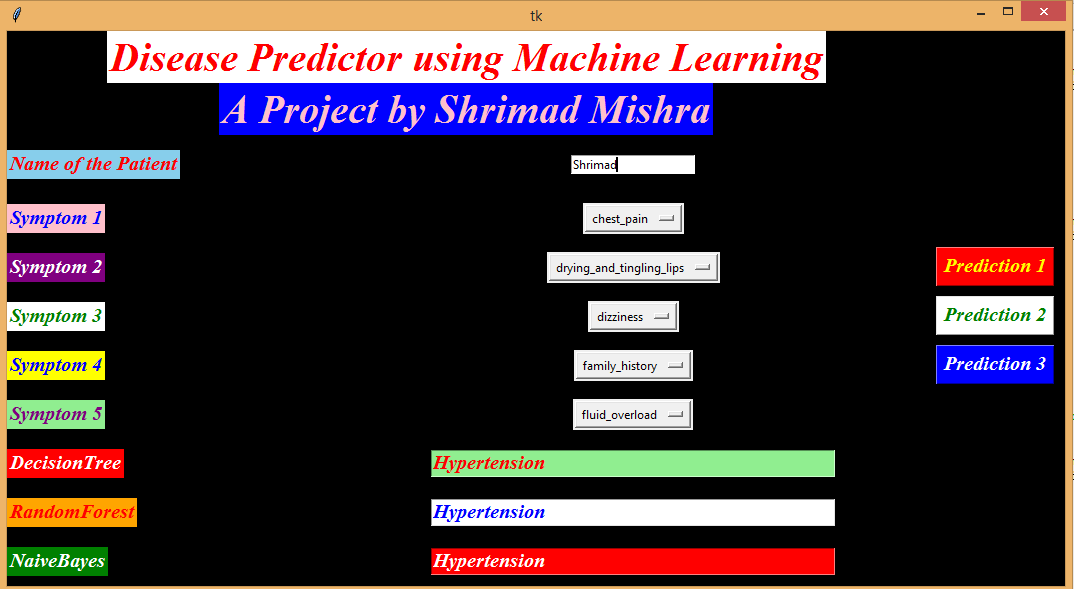# Disease Prediction Using Machine Learning In Python Using GUI

Hi, guys Today We will do a project which will predict the disease by taking symptoms from the user.

Let us start the project, we will learn about the three different algorithms in machine learning.

The first algorithm is a Decision Tree, second is a Random Forest and the last one is Naive Bayes.

We are going to import Pandas for manipulating the CSV file, Numpy, Sklearn for the algorithms and  Tkinter for our GUI stuff.

Because If we use a single algorithm for our project then how we come to know that the prediction is correct.

So that’s why we use three algorithms.

Now our first step is to make a list or dataset of the symptoms and diseases.

The dataset is given below:

Prototype.csv

Prototype1.csv

## Disease Prediction GUI Project In Python Using ML

```from tkinter import *
import numpy as np
import pandas as pd

#List of the symptoms is listed here in list l1.

l1=['back_pain','constipation','abdominal_pain','diarrhoea','mild_fever','yellow_urine',
'swelled_lymph_nodes','malaise','blurred_and_distorted_vision','phlegm','throat_irritation',
'redness_of_eyes','sinus_pressure','runny_nose','congestion','chest_pain','weakness_in_limbs',
'fast_heart_rate','pain_during_bowel_movements','pain_in_anal_region','bloody_stool',
'irritation_in_anus','neck_pain','dizziness','cramps','bruising','obesity','swollen_legs',
'swollen_blood_vessels','puffy_face_and_eyes','enlarged_thyroid','brittle_nails',
'swollen_extremeties','excessive_hunger','extra_marital_contacts','drying_and_tingling_lips',
'slurred_speech','knee_pain','hip_joint_pain','muscle_weakness','stiff_neck','swelling_joints',
'continuous_feel_of_urine','passage_of_gases','internal_itching','toxic_look_(typhos)',
'depression','irritability','muscle_pain','altered_sensorium','red_spots_over_body','belly_pain',
'abnormal_menstruation','dischromic _patches','watering_from_eyes','increased_appetite','polyuria','family_history','mucoid_sputum',
'rusty_sputum','lack_of_concentration','visual_disturbances','receiving_blood_transfusion',
'receiving_unsterile_injections','coma','stomach_bleeding','distention_of_abdomen',
'silver_like_dusting','small_dents_in_nails','inflammatory_nails','blister','red_sore_around_nose',
'yellow_crust_ooze']

#List of Diseases is listed in list disease.

disease=['Fungal infection','Allergy','GERD','Chronic cholestasis','Drug Reaction',
'Peptic ulcer diseae','AIDS','Diabetes','Gastroenteritis','Bronchial Asthma','Hypertension',
' Migraine','Cervical spondylosis',
'Paralysis (brain hemorrhage)','Jaundice','Malaria','Chicken pox','Dengue','Typhoid','hepatitis A',
'Hepatitis B','Hepatitis C','Hepatitis D','Hepatitis E','Alcoholic hepatitis','Tuberculosis',
'Common Cold','Pneumonia','Dimorphic hemmorhoids(piles)',
'Heartattack','Varicoseveins','Hypothyroidism','Hyperthyroidism','Hypoglycemia','Osteoarthristis',
'Arthritis','(vertigo) Paroymsal  Positional Vertigo','Acne','Urinary tract infection','Psoriasis',
'Impetigo']

l2=[]

for i in range(0,len(l1)):
l2.append(0)```

Now the main part of machine learning comes here i.e the training and testing of the code or model.

So the training file is named as prototype.csv in our program and the testing file is named as prototype 1.csv.

In the last of the article, there is a link to the files.

Import those files by using pandas and replace the items in the files as shown in the code.

Traverse the file as shown in the code and store them into an x_test and y_test. Then  Ravel the y_text using the Numpy module.

```df=pd.read_csv("Prototype.csv")

#Replace the values in the imported file by pandas by the inbuilt function replace in pandas.

df.replace({'prognosis':{'Fungal infection':0,'Allergy':1,'GERD':2,'Chronic cholestasis':3,'Drug Reaction':4,
'Peptic ulcer diseae':5,'AIDS':6,'Diabetes ':7,'Gastroenteritis':8,'Bronchial Asthma':9,'Hypertension ':10,
'Migraine':11,'Cervical spondylosis':12,
'Paralysis (brain hemorrhage)':13,'Jaundice':14,'Malaria':15,'Chicken pox':16,'Dengue':17,'Typhoid':18,'hepatitis A':19,
'Hepatitis B':20,'Hepatitis C':21,'Hepatitis D':22,'Hepatitis E':23,'Alcoholic hepatitis':24,'Tuberculosis':25,
'Common Cold':26,'Pneumonia':27,'Dimorphic hemmorhoids(piles)':28,'Heart attack':29,'Varicose veins':30,'Hypothyroidism':31,
'Hyperthyroidism':32,'Hypoglycemia':33,'Osteoarthristis':34,'Arthritis':35,
'(vertigo) Paroymsal  Positional Vertigo':36,'Acne':37,'Urinary tract infection':38,'Psoriasis':39,
'Impetigo':40}},inplace=True)

#check the df

X= df[l1]

#print(X)

y = df[["prognosis"]]
np.ravel(y)

#print(y)

#Use replace method in pandas.

tr.replace({'prognosis':{'Fungal infection':0,'Allergy':1,'GERD':2,'Chronic cholestasis':3,'Drug Reaction':4,
'Peptic ulcer diseae':5,'AIDS':6,'Diabetes ':7,'Gastroenteritis':8,'Bronchial Asthma':9,'Hypertension ':10,
'Migraine':11,'Cervical spondylosis':12,
'Paralysis (brain hemorrhage)':13,'Jaundice':14,'Malaria':15,'Chicken pox':16,'Dengue':17,'Typhoid':18,'hepatitis A':19,
'Hepatitis B':20,'Hepatitis C':21,'Hepatitis D':22,'Hepatitis E':23,'Alcoholic hepatitis':24,'Tuberculosis':25,
'Common Cold':26,'Pneumonia':27,'Dimorphic hemmorhoids(piles)':28,'Heart attack':29,'Varicose veins':30,'Hypothyroidism':31,
'Hyperthyroidism':32,'Hypoglycemia':33,'Osteoarthristis':34,'Arthritis':35,
'(vertigo) Paroymsal  Positional Vertigo':36,'Acne':37,'Urinary tract infection':38,'Psoriasis':39,
'Impetigo':40}},inplace=True)

X_test= tr[l1]
y_test = tr[["prognosis"]]

#print(y_test)

np.ravel(y_test)```

Now here comes the algorithm part of our program.

Before going through the algorithms, I advise you to go through the algorithms we are going to use in this project.

After understanding the basics of algorithms now apply them in our project.

If the prediction by any of the two algorithms is the same the user might have that disease.

But all the algorithms predict different diseases then the user is trying to make the machine fool.

```def DecisionTree():

from sklearn import tree

clf3 = tree.DecisionTreeClassifier()
clf3 = clf3.fit(X,y)

from sklearn.metrics import accuracy_score
y_pred=clf3.predict(X_test)
print(accuracy_score(y_test, y_pred))
print(accuracy_score(y_test, y_pred,normalize=False))

psymptoms = [Symptom1.get(),Symptom2.get(),Symptom3.get(),Symptom4.get(),Symptom5.get()]

for k in range(0,len(l1)):
for z in psymptoms:
if(z==l1[k]):
l2[k]=1

inputtest = [l2]
predict = clf3.predict(inputtest)
predicted=predict

h='no'
for a in range(0,len(disease)):
if(predicted == a):
h='yes'
break

if (h=='yes'):
t1.delete("1.0", END)
t1.insert(END, disease[a])
else:
t1.delete("1.0", END)

def randomforest():
from sklearn.ensemble import RandomForestClassifier
clf4 = RandomForestClassifier()
clf4 = clf4.fit(X,np.ravel(y))

# calculating accuracy
from sklearn.metrics import accuracy_score
y_pred=clf4.predict(X_test)
print(accuracy_score(y_test, y_pred))
print(accuracy_score(y_test, y_pred,normalize=False))

psymptoms = [Symptom1.get(),Symptom2.get(),Symptom3.get(),Symptom4.get(),Symptom5.get()]

for k in range(0,len(l1)):
for z in psymptoms:
if(z==l1[k]):
l2[k]=1

inputtest = [l2]
predict = clf4.predict(inputtest)
predicted=predict

h='no'
for a in range(0,len(disease)):
if(predicted == a):
h='yes'
break

if (h=='yes'):
t2.delete("1.0", END)
t2.insert(END, disease[a])
else:
t2.delete("1.0", END)

def NaiveBayes():
from sklearn.naive_bayes import GaussianNB
gnb = GaussianNB()
gnb=gnb.fit(X,np.ravel(y))

from sklearn.metrics import accuracy_score
y_pred=gnb.predict(X_test)
print(accuracy_score(y_test, y_pred))
print(accuracy_score(y_test, y_pred,normalize=False))

psymptoms = [Symptom1.get(),Symptom2.get(),Symptom3.get(),Symptom4.get(),Symptom5.get()]
for k in range(0,len(l1)):
for z in psymptoms:
if(z==l1[k]):
l2[k]=1

inputtest = [l2]
predict = gnb.predict(inputtest)
predicted=predict

h='no'
for a in range(0,len(disease)):
if(predicted == a):
h='yes'
break

if (h=='yes'):
t3.delete("1.0", END)
t3.insert(END, disease[a])
else:
t3.delete("1.0", END)
```

Now we have done with all the computation part.

We are going to use Tkinter for our GUI because of different IDE. In Anaconda we can use a simple drag and drop.

But in other IDE we have to write the big codes.

Now design the GUI for our project.

```# GUI stuff..............................................................................

root = Tk()
root.configure(background='black')

Symptom1 = StringVar()
Symptom1.set("Select Here")

Symptom2 = StringVar()
Symptom2.set("Select Here")

Symptom3 = StringVar()
Symptom3.set("Select Here")

Symptom4 = StringVar()
Symptom4.set("Select Here")

Symptom5 = StringVar()
Symptom5.set("Select Here")

Name = StringVar()

w2 = Label(root, justify=LEFT, text="Disease Predictor using Machine Learning", fg="Red", bg="White")
w2.config(font=("Times",30,"bold italic"))
w2 = Label(root, justify=LEFT, text="A Project by Shrimad Mishra", fg="Pink", bg="Blue")
w2.config(font=("Times",30,"bold italic"))

NameLb = Label(root, text="Name of the Patient", fg="Red", bg="Sky Blue")
NameLb.config(font=("Times",15,"bold italic"))

S1Lb = Label(root, text="Symptom 1", fg="Blue", bg="Pink")
S1Lb.config(font=("Times",15,"bold italic"))

S2Lb = Label(root, text="Symptom 2", fg="White", bg="Purple")
S2Lb.config(font=("Times",15,"bold italic"))

S3Lb = Label(root, text="Symptom 3", fg="Green",bg="white")
S3Lb.config(font=("Times",15,"bold italic"))

S4Lb = Label(root, text="Symptom 4", fg="blue", bg="Yellow")
S4Lb.config(font=("Times",15,"bold italic"))

S5Lb = Label(root, text="Symptom 5", fg="purple", bg="light green")
S5Lb.config(font=("Times",15,"bold italic"))

lrLb = Label(root, text="DecisionTree", fg="white", bg="red")
lrLb.config(font=("Times",15,"bold italic"))

destreeLb = Label(root, text="RandomForest", fg="Red", bg="Orange")
destreeLb.config(font=("Times",15,"bold italic"))

ranfLb = Label(root, text="NaiveBayes", fg="White", bg="green")
ranfLb.config(font=("Times",15,"bold italic"))

OPTIONS = sorted(l1)

NameEn = Entry(root, textvariable=Name)
NameEn.grid(row=6, column=1)

S1.grid(row=7, column=1)

S2.grid(row=8, column=1)

S3.grid(row=9, column=1)

S4.grid(row=10, column=1)

S5.grid(row=11, column=1)

dst = Button(root, text="Prediction 1", command=DecisionTree,bg="Red",fg="yellow")
dst.config(font=("Times",15,"bold italic"))

rnf = Button(root, text="Prediction 2", command=randomforest,bg="White",fg="green")
rnf.config(font=("Times",15,"bold italic"))

lr = Button(root, text="Prediction 3", command=NaiveBayes,bg="Blue",fg="white")
lr.config(font=("Times",15,"bold italic"))

t1 = Text(root, height=1, width=40,bg="Light green",fg="red")
t1.config(font=("Times",15,"bold italic"))

t2 = Text(root, height=1, width=40,bg="White",fg="Blue")
t2.config(font=("Times",15,"bold italic"))

t3 = Text(root, height=1, width=40,bg="red",fg="white")
t3.config(font=("Times",15,"bold italic"))

root.mainloop()```

Here is the output### 21 responses to “Disease Prediction Using Machine Learning In Python Using GUI”

1. Honey says:

Really an amazing and wonderful project…. you deserve the appreciation. Keep the same spirit brother. Hope it would help countless people in future.

2. Pushp says:

Thanks Sir. It is easy to understand and very helpful for beginners.

3. Sarth says:

Amazing stuff. Very well coded .

4. Vinay says:

Very good initiative

5. Gopi Raman Mishra says:

Really marvellous work. GUI is easy to focus and very complex. It is really helpful for New programmers. Well done. Thumbs up.

6. jeffrey says:

where is the prototype file!! unable to find it… ….

7. jeffrey says:

8. Rohan agrawal says:

Can you provide the link for prototype.csv and prototype 1.csv datasets?

9. Itishree says:

Only prototype.csv is there.where is prototype 1.csv ?

10. shivang saxena says:

where is the prototype1.csv file please provide the link of that file so we can proceed further.

11. Yash Vibhute says:

Exception in Tkinter callback
Traceback (most recent call last):
File “C:\Users\Yash\AppData\Local\Programs\Python\Python38\lib\tkinter\__init__.py”, line 1883, in __call__
return self.func(*args)
File “C:/Users/Yash/PycharmProjects/pythonProject1/codeyash.py”, line 42, in randomforest
clf4 = clf4.fit(X,np.ravel(y))
File “C:\Users\Yash\AppData\Local\Programs\Python\Python38\lib\site-packages\sklearn\ensemble\_forest.py”, line 330, in fit
y, expanded_class_weight = self._validate_y_class_weight(y)
File “C:\Users\Yash\AppData\Local\Programs\Python\Python38\lib\site-packages\sklearn\ensemble\_forest.py”, line 558, in _validate_y_class_weight
check_classification_targets(y)
File “C:\Users\Yash\AppData\Local\Programs\Python\Python38\lib\site-packages\sklearn\utils\multiclass.py”, line 172, in check_classification_targets
raise ValueError(“Unknown label type: %r” % y_type)
ValueError: Unknown label type: ‘unknown’